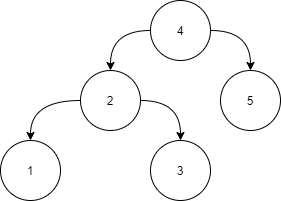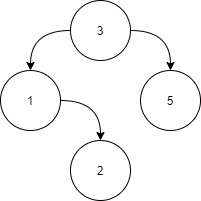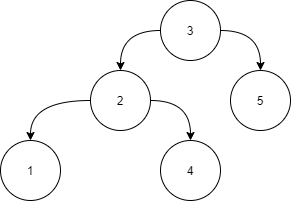New update is available. Click here to update.Close
Topic list
Partial BST
MEDIUM
25 mins45 upvotes
Binary Search Trees
Trees
Topics (Covered in this problem)
Problem solved
Skill meterTrees
-
-Binary Search Trees
-
-
Other topics
Problem solved
Skill meterStrings
-
-Matrices (2D Arrays)
-
--
-Sorting
-
-Binary Search
-
-Stacks & Queues
-
-Graph
-
-Dynamic Programming
-
-Greedy
-
-Tries
-
-Arrays
-
-SQL
-
-Heap
-
-Bit Manipulation
-
-
Solve problems & track your progress
Checkout your overall progress in every topic here
BecomeSensei
in DSA topics
Open the topic and solve more problems associated with it to improve your skills
Check out the skill meter for every topic
See how many problems you are left with to solve for cracking any stage. Score more than zero to get your progress counted.

# Partial BST

Medium0/80
Avg time to solve 25 mins
Success Rate 70 %Share45 upvotes

## Problem Statement

#### A binary search tree (BST) is said to be a Partial BST if it follows the following properties.

``````• The left subtree of a node contains only nodes with data less than and equal to the node’s data.
• The right subtree of a node contains only nodes with data greater than and equal to the node’s data.
• Both the left and right subtrees must also be partial binary search trees.
``````

#### Input:``````Level 1:

All the nodes in the left subtree of 4 (2, 1, 3) are smaller
than 4, all the nodes in the right subtree of the 4 (5) are
larger than 4.

Level 2 :

For node 2:
All the nodes in the left subtree of 2 (1) are smaller than
2, all the nodes in the right subtree of the 2 (3) are larger than 2.
For node 5:
The left and right subtree for node 5 is empty.

Level 3:

For node 1:
The left and right subtree for node 1 are empty.
For node 3:
The left and right subtree for node 3 are empty.
Because all the nodes follow the property of a Partial binary
search tree, the above tree is a Partial binary search tree.
``````
Detailed explanation ( Input/output format, Notes, Constraints, Images )##### Sample Input 1:
``````2
3 1 5 -1 2 -1 -1 -1 -1
3 2 5 1 4 -1 -1 -1 -1 -1 -1
``````
##### Sample Output 1:
`````` true
false
``````
##### Explanation of the Sample Input1:
``````Here we have 2 test cases, hence there are 2 binary trees

Test Case 1:
```````````` Level 1:
For node 3 all the nodes in the left subtree (1,2) are
less than 3 and all the nodes in the right subtree (5)
are greater than 3.

Level 2:
For node 1:
The left subtree is empty and all the nodes in the right
subtree (2) are greater than 1.
For node 5:
Both right and left subtrees are empty.

Level 3:
For node 2, both right and left subtrees are empty.
Because all the nodes follow the property of a Partial binary
search tree, the function should return true.

Test Case 2:
````````````For the root node, all the nodes in the right subtree (5) are greater than 3. But node with data 4 in the left subtree of node 3 is greater than 3, this does not satisfy the condition for the Partial binary search tree. Hence, the function should return false.
``````AutoConsole